Next: About this document ... Up: lab_template Previous: lab_template

Subsections

# MA 1024 Lab 3: Global Extrema

## Purpose

The purpose of this lab is to acquaint you with techniques for finding global extreme values of functions of two variables.

## Getting Started

To assist you, there is a worksheet associated with this lab that contains examples and even solutions to some of the exercises. You can copy that worksheet to your home directory by going to your computer's Start menu and choose run. In the run field type:

\\filer\calclab


when you hit enter, you can then choose MA1024 and then choose the worksheet

Optimization_start_C14.mw


Remember to immediately save it in your own home directory. Once you've copied and saved the worksheet, read through the background on the internet and the background of the worksheet before starting the exercises.

## Background

Many applications of calculus involve finding the maximum and minimum values of functions. For example, suppose that there is a network of electrical power generating stations, each with its own cost for producing power, with the cost per unit of power at each station changing with the amount of power it generates. An important problem for the network operators is to determine how much power each station should generate to minimize the total cost of generating a given amount of power.

A crucial first step in solving such problems is being able to find and classify local extreme values of a function. What we mean by a function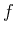having a local extreme value at a pointis that for values of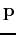near,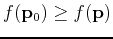for a local maximum andfor a local minimum.

In single-variable calculus, we found that we could locate candidates for local extreme values by finding points where the first derivative vanishes. For functions of two dimensions, the condition is that both first order partial derivatives must vanish at a local extreme value candidate point. Such a point is called a stationary point. It is also one of the three types of points called critical points. Note carefully that the condition does not say that a point where the partial derivatives vanish must be a local extreme point. Rather, it says that stationary points are candidates for local extrema. Just as was the case for functions of a single variable, there can be stationary points that are not extrema. For example, the saddle surface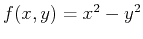has a stationary point at the origin, but it is not a local extremum.

Finding and classifying the local extreme values of a function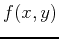requires several steps. First, the partial derivatives must be computed. Then the stationary points must be solved for by finding where both first partial derivatives are zero simultaneously, which is not always a simple task. Next, one must check for the presence of singular points, which might also be local extreme values. Finally, each critical point must be classified as a local maximum, local minimum, or neither. The examples in the Getting Started worksheet are intended to help you learn how to use Maple to simplify these tasks.

### Locating Global Extrema

In one-dimensional calculus, the absolute or global extreme values of a function occur either at a point where the derivative is zero, a boundary point, or where the derivative fails to exist. The situation for a function of two variables is very similar, but the problem is much more difficult because the boundary now consists of curves instead of just endpoints of intervals. For example, suppose that we wanted to find the global extreme values of a functionon the rectangle. The boundary of this rectangle consists of the four line segments given below.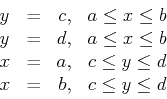The basic theorem on the existence of global maximum and minimum values is the following.

Theorem 1   Supposeis continuous on a closed, bounded set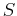, thenattains its absolute maximum value at some pointinand absolute minimum value at some pointin.

This theorem only says that the extrema exist, but doesn't help at all in finding them. However, we know that the global extrema occur either at local extrema, on the boundary of the region, or at points where one or the other partial derivative fails to exist. For example, to find the extreme values of a functionon the rectangle given above, you would first have to find the interior critical points and then find the extreme values for the four one-dimensional functions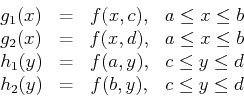## Exercises

1. Consider the function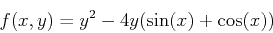Find the absolute extrema of this function over the region bounded by the rectangle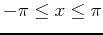,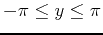.

2. Find and classify the global extrema for the functionon the region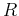bounded by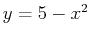and.Next: About this document ... Up: lab_template Previous: lab_template
Dina J. Solitro-Rassias
2014-02-03